Updating search results...

# 20 Results

View
Selected filters:
• Learnzillion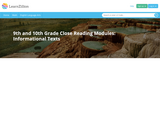Restricted Use
Rating
0.0 stars

This resource is a multi-day lesson plan that guides students through the close reading process of an informational text. Using the 1941 FDR State of the Union address, components of informational text including: organization, context, and rhetoric are analyzed. This resource combines lessons plans, primary text, read aloud of the text, informational video, and text complexity / vocabulary Analysis.

Subject:
Civics and Government
Social Studies
Material Type:
Lesson Plan
Primary Source
Provider:
Learnzillion
12/28/2015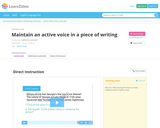Restricted Use
Rating
0.0 stars

This video shares how a writer can keep an active voice within a piece of writing. There are places for the viewers to stop and practice together prior to moving on. A student practice sheet is available as a download for additional practice.

Subject:
English Language Arts
Material Type:
Formative Assessment
Lesson Plan
Self Assessment
Simulation
Provider:
Learnzillion
04/19/2016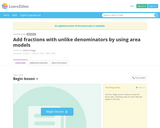Restricted Use
Rating
0.0 stars

This resource can be used to present to students how to add fractions with  unlike denominators by using area models to represent the fractions.

Subject:
Mathematics
Material Type:
Lesson Plan
Provider:
Learnzillion
06/16/2015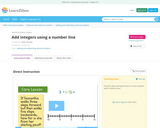Rating
0.0 stars

This resource is a teaching video for students to watch to learn how to add integers using a number line.  The video relates adding integers to real world problems during the lessons.

Subject:
Mathematics
Material Type:
Diagram/Illustration
Simulation
Provider:
Learnzillion
03/20/2018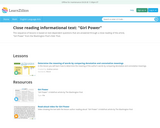Rating
0.0 stars

This sequence of lessons is based on text-dependent questions that are answered through a close reading of the article, "Girl Power" from the Washington Post's Kids' Post.

Subject:
English Language Arts
Material Type:
Lesson Plan
Unit of Study
Provider:
Learnzillion
03/20/2018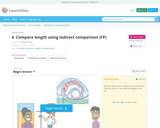Rating
0.0 stars

Lesson objective: Compare lengths of objects both directly and indirectly. Understand that if ​object A is longer than object B and object B is longer than object C, then object A is longer than object C.
This lesson helps to build fluency with comparing lengths of objects indirectly. Images and clues are used here because they allow students to build the relationships between direct and indirect comparison.
Students engage in Mathematical Practice 7 (Look for and make use of structure) as they use transitivity, indirect comparison to compare length measurement.

Subject:
Mathematics
Material Type:
Lesson Plan
Provider:
Learnzillion
03/20/2018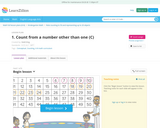Rating
0.0 stars

In this lesson students will understand that they can begin counting at a number other than 1 in a counting sequence. Students will understand that numbers follow a sequence. this will help students gain an understanding of operations as they learn to count on from any number they will develop strategies for counting on in addition.

Subject:
Mathematics
Material Type:
Game
Lesson Plan
Provider:
Learnzillion
03/20/2018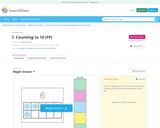Rating
0.0 stars

This lesson will build fluency with counting objects to 10. Students will engage in counting with objects and a 10-frame to recognize groups of numbers.

Subject:
Mathematics
Material Type:
Game
Lesson Plan
Provider:
Learnzillion
03/20/2018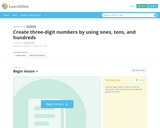Conditional Remix & Share Permitted
CC BY-NC-SA
Rating
0.0 stars

Big Idea: 3-digit numbers can contain a Ã¢â‚¬Å“hundred," which is equal to 10 groups of 10.
This lesson builds on previous lessons' work with two-digit numbers. The task asks students to model the three-digit number shown in the question according to guidelines, i.e. with only hundreds and tens. This approach gives students support in building three-digit numbers; the base ten blocks give students a jumping-off point for their thinking. For instance, they can immediately think about how they could show 21 in only ones instead of tens and ones.
Special Materials: Base Ten Blocks

Subject:
Mathematics
Material Type:
Assessment Item
Diagram/Illustration
Game
Lesson Plan
Provider:
Learnzillion
03/08/2017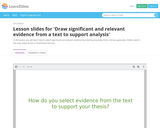Restricted Use
Rating
0.0 stars

This LearnZillion video models how to select significant and relevant evidence by selecting examples from a written text. The video reviews the writing process and provides an example thesis based on "An Occurrence at Owl Creek Bridge" and the development of concurrent themes. The process of choosing pieces of texual evidence that best support the thesis will be modeled.

Subject:
English Language Arts
Material Type:
Assessment Item
Diagram/Illustration
Lesson Plan
Simulation
Provider:
Learnzillion
11/03/2015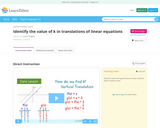Rating
0.0 stars

This activity clearly shows students how to find the value of k in a traanslation of a linear function.  It shows the student the difference between a vertical and a horizontal translation.

Subject:
Mathematics
Material Type:
Lesson Plan
Simulation
Provider:
Learnzillion
03/20/2018Conditional Remix & Share Permitted
CC BY-NC-SA
Rating
0.0 stars

Big Ideas: The same number can be shown in many different ways. 10 bundles of 10 can be combined to make a Ã¢â‚¬Å“hundred."
This lesson introduces the concept of "hundreds" and the various ways that one hundred can be made using groups of tens and ones. The task presents a basketball game in which the points are base ten values. The students are asked to think about and represent the different ways one could score 100 points. Understanding that there is no one "right" way to represent a number using base ten helps students have a solid foundation for later skills such as place value of 3 and 4 digit numbers, addition and subtraction with regrouping, and multiplication.
Special Materials: Base Ten Blocks

Subject:
Mathematics
Material Type:
Assessment Item
Game
Lesson Plan
Provider:
Learnzillion
03/08/2017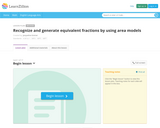Restricted Use
Rating
0.0 stars

This lesson builds on students' work explaining equivalence of fractions in special cases and comparing fractions by reasoning about their size. The task uses area models to demonstrate the equivalence of fractions. This builds toward future work with understanding addition and subtraction of fractions as joining and separating parts referring to the same whole.

Subject:
Mathematics
Material Type:
Lesson Plan
Provider:
Learnzillion
12/28/2015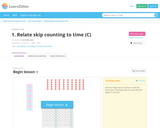Restricted Use
Rating
0.0 stars

In this unit, students integrate their understanding of skip counting by 5s and 10s to support telling and writing time to the nearest five minutes. They expand their prior knowledge of telling time to the nearest half hour, hour, and counting by tens to include counting by fives. Applying the knowledge of counting by fives and tens to the clock equips students to be successful in the real-life situation of telling time.

Subject:
Mathematics
Material Type:
Activity/Lab
Full Course
Interactive
Lesson Plan
Unit of Study
Provider:
Learnzillion
04/05/2016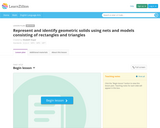Restricted Use
Rating
0.0 stars

This resource allows students to build conceptual relationships between two-dimensional shapes and three-dimensional figures by means of nets. Students cut different nets and identify and describe the corresponding figures. Students realize and understand why surface areas are measured in square units, and volume is measured in cubic units.

Subject:
Mathematics
Material Type:
Curriculum Map
Lesson Plan
Provider:
Learnzillion
12/28/2015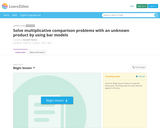Restricted Use
Rating
0.0 stars

This lesson builds on students' understanding of multiplicative comparison. The task asks students to determine the height of a landmark structure in comparison to the given height of an ancient structure. Students will problem solve and use various mathematical models to solve a multiplicative comparison with an unknown product. This builds toward using equations with a symbol for the unknown to solve word problems with an unknown product. This lesson supports the 4th grade standard of solving word problems involving multiplicative comparison by using drawings and equations.

Subject:
Mathematics
Material Type:
Lesson Plan
Provider:
Learnzillion
12/28/2015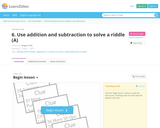Restricted Use
Rating
0.0 stars

This lesson provides an opportunity for students to apply their knowledge and understanding of adding and subtracting two digit numbers to a mathematical situation. Students are asked to use clue cards to determine how many exercises should be completed

Subject:
Mathematics
Material Type:
Activity/Lab
Assessment Item
Diagram/Illustration
Formative Assessment
Game
Interactive
Lesson Plan
Self Assessment
Simulation
Teaching/Learning Strategy
Unit of Study
Provider:
Learnzillion
04/05/2016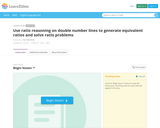Restricted Use
Rating
0.0 stars

In this lesson, students will use double number lines and ratio reasoning to solve ratio problems. By partitioning double number lines into proportionate intervals, students will build an understanding of equivalent ratio relationships as those that are generated by multiplying or dividing two related quantities by the same number. This lesson will build upon students' prior understanding of multiplicative comparisons, number line reasoning and equivalent fractions (while highlighting the difference between equivalent fractions and equivalent ratios); and will build toward future work in this unit on building ratio tables, and work in future grades in proportionality, scaling, and expressing and graphing linear functions.

Subject:
Mathematics
Material Type:
Lesson Plan
Provider:
Learnzillion
12/28/2015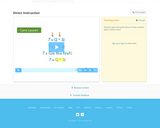Rating
0.0 stars

This resource can be used when teaching students how to evaluate expressions that have parentheses. The resource includes a short (5min) video as well as a 12 slide presentation that could be used in a number of ways. The video could be viewed by students as a preview to the lesson, during the lesson, or after the lesson as a review. It also could be used as a remediation tool for students who are struggling with parenthese use in mathematics. The slide presentation could be used by the teacher as a lesson guide.

Subject:
Mathematics
Material Type:
Diagram/Illustration
Lesson Plan
Simulation
Provider:
Learnzillion
03/20/2018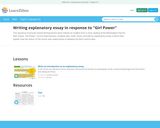Rating
0.0 stars

This sequence of process-based writing lessons leans heavily on insights from a close reading of the Washington Post for Kids' article, "Girl Power." Across these lessons, students plan, draft, revise, and edit an explanatory essay in which they explain how the author of the article uses relationships to develop the text's central idea.

Subject:
English Language Arts
Material Type:
Lesson Plan